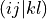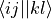# DataHfProvider¶

class adcc.DataHfProvider(data)

Methods Summary

 fill_eri_ffff(self, arg0, arg1) Fill the passed numpy array arg1 with a part of the electron-repulsion integral tensor in the molecular orbital basis. fill_eri_phys_asym_ffff(self, arg0, arg1) Fill the passed numpy array arg1 with a part of the antisymmetrised electron-repulsion integral tensor in the molecular orbital basis. fill_fock_ff(self, arg0, arg1) Fill the passed numpy array arg1 with a part of the Fock matrix in the molecular orbital basis. fill_occupation_f(self, arg0) Fill the passed numpy array of size (2 * nf, ) with the occupation number for each SCF orbital. fill_orbcoeff_fb(self, arg0) Fill the passed numpy array of size (2 * nf, nb) with the SCF orbital coefficients, i.e. fill_orben_f(self, arg0) Fill the passed numpy array of size (2 * nf, ) with the SCF orbital energies. get_backend() get_conv_tol(self) Returns the tolerance value used for SCF convergence. get_energy_scf(self) Returns the final total SCF energy (sum of electronic and nuclear terms. get_n_bas(self) Returns the number of spatial one-electron basis functions. get_n_orbs_alpha(self) Returns the number of HF spin orbitals of alpha spin. get_nuclear_multipole(self, arg0) Returns the nuclear multipole of the requested order. get_restricted(self) Return True for a restricted SCF calculation, False otherwise. get_spin_multiplicity(self) Returns the spin multiplicity of the HF ground state. has_eri_phys_asym_ffff_inner()

Methods Documentation

fill_eri_ffff(self: libadcc.HartreeFockProvider, arg0: tuple, arg1: array) → None

Fill the passed numpy array arg1 with a part of the electron-repulsion integral tensor in the molecular orbital basis. The indexing convention is the chemist’s notation, i.e. the index tuple (i,j,k,l) refers to the integral. The block to store is specified by the provided tuple of ranges arg0, which gives the range of indices to place into the buffer along each of the axis. The index counting is done in spin orbitals, so the full range in each axis is range(0, 2 * nf).

fill_eri_phys_asym_ffff(self: libadcc.HartreeFockProvider, arg0: tuple, arg1: array) → None

Fill the passed numpy array arg1 with a part of the antisymmetrised electron-repulsion integral tensor in the molecular orbital basis. The indexing convention is the physicist’s notation, i.e. the index tuple (i,j,k,l) refers to the integral. The block to store is specified by the provided tuple of ranges arg0, which gives the range of indices to place into the buffer along each of the axis. The index counting is done in spin orbitals, so the full range in each axis is range(0, 2 * nf).

fill_fock_ff(self: libadcc.HartreeFockProvider, arg0: tuple, arg1: array) → None

Fill the passed numpy array arg1 with a part of the Fock matrix in the molecular orbital basis. The block to store is specified by the provided tuple of ranges arg0, which gives the range of indices to place into the buffer along each of the axis. The index counting is done in spin orbitals, so the full range in each axis is range(0, 2 * nf). The implementation should not assume that the alpha-beta and beta-alpha blocks are not accessed even though they are zero by spin symmetry.

fill_occupation_f(self: libadcc.HartreeFockProvider, arg0: array) → None

Fill the passed numpy array of size (2 * nf, ) with the occupation number for each SCF orbital.

fill_orbcoeff_fb(self: libadcc.HartreeFockProvider, arg0: array) → None

Fill the passed numpy array of size (2 * nf, nb) with the SCF orbital coefficients, i.e. the uniform transform from the one-particle basis to the molecular orbitals.

fill_orben_f(self: libadcc.HartreeFockProvider, arg0: array) → None

Fill the passed numpy array of size (2 * nf, ) with the SCF orbital energies.

get_backend()
get_conv_tol(self: libadcc.HartreeFockProvider) → float

Returns the tolerance value used for SCF convergence. Should be roughly equivalent to the l2 norm of the Pulay error.

get_energy_scf(self: libadcc.HartreeFockProvider) → float

Returns the final total SCF energy (sum of electronic and nuclear terms.

get_n_bas(self: libadcc.HartreeFockProvider) → int

Returns the number of spatial one-electron basis functions. This value is abbreviated by nb in the documentation.

get_n_orbs_alpha(self: libadcc.HartreeFockProvider) → int

Returns the number of HF spin orbitals of alpha spin. It is assumed the same number of beta spin orbitals are used. This value is abbreviated by nf in the documentation.

get_nuclear_multipole(self: libadcc.HartreeFockProvider, arg0: int) → numpy.ndarray[float64]

Returns the nuclear multipole of the requested order. For 0 returns the total nuclear charge as an array of size 1, for 1 returns the nuclear dipole moment as an array of size 3.

get_restricted(self: libadcc.HartreeFockProvider) → bool

Return True for a restricted SCF calculation, False otherwise.

get_spin_multiplicity(self: libadcc.HartreeFockProvider) → int

Returns the spin multiplicity of the HF ground state. A value of 0* (for unknown) should be supplied for unrestricted calculations.

has_eri_phys_asym_ffff_inner()